# Set Theory Symbols

Symbol
Meaning / definition
Example
{ }
a collection of elements
A = {3,7,9,14},
B = {9,14,28}
A ∩ B
objects that belong to set A and set B
A ∩ B = {9,14}
A ∪ B
objects that belong to set A or set B
A ∪ B = {3,7,9,14,28}
A ⊆ B
subset has fewer elements or equal to the set
{9,14,28} ⊆ {9,14,28}
A ⊂ B
subset has fewer elements than the set
{9,14} ⊂ {9,14,28}
A ⊄ B
left set not a subset of right set
{9,66} ⊄ {9,14,28}
A ⊇ B
set A has more elements or equal to the set B
{9,14,28} ⊇ {9,14,28}
A ⊃ B
set A has more elements than set B
{9,14,28} ⊃ {9,14}
A ⊅ B
set A is not a superset of set B
{9,14,28} ⊅ {9,66}
2power set
all subsets of A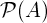all subsets of A
A = B
both sets have the same members
A={3,9,14},
B={3,9,14},
A=B
Ac
all the objects that do not belong to set A
A \ B
objects that belong to A and not to B
A = {3,9,14},
B = {1,2,3},
A-B = {9,14}
A – B
objects that belong to A and not to B
A = {3,9,14},
B = {1,2,3},
A-B = {9,14}
A ∆ B
objects that belong to A or B but not to their intersection
A = {3,9,14},
B = {1,2,3},
A ∆ B = {1,2,9,14}
A ⊖ B
objects that belong to A or B but not to their intersection
A = {3,9,14},
B = {1,2,3},
A ⊖ B = {1,2,9,14}
a∈A
set membership
A={3,9,14}, 3 ∈ A
x∉A
no set membership
A={3,9,14}, 1 ∉ A
(a,b)
collection of 2 elements
A×B
set of all ordered pairs from A and B
|A|
the number of elements of set A
A={3,9,14}, |A|=3
#A
the number of elements of set A
A={3,9,14}, #A=3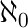infinite cardinality of natural numbers set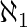cardinality of countable ordinal numbers set
Ø
Ø = { }
C = {Ø}set of all possible values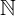00 = {0,1,2,3,4,…}
0 ∈011 = {1,2,3,4,5,…}
6 ∈1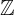= {…-3,-2,-1,0,1,2,3,…}
-6 ∈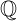= {x | x=a/b, a,b}
2/6 ∈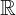= {x | -∞ < x <∞}
6.343434 ∈= {z | z=a+bi, -∞<a<∞, -∞<b<∞}
6+2i